## 28 August 2018

### testing syntax

``` <script type='text/javascript'>
//<![CDATA[
(function(d, s, id) {
var js, fjs = d.getElementsByTagName(s);
if (d.getElementById(id)) return;
js = d.createElement(s); js.id = id;
js.src = "//connect.facebook.net/en_US/all.js#xfbml=1&appId=App-ID";
fjs.parentNode.insertBefore(js, fjs);
}(document, 'script', 'facebook-jssdk'));
//]]>
</script>
```

## 10 August 2018

### Deep Learning : Neural Network, Feed Forward, Activation Function, Back Propagation, Stochastic Gradient Descent

Deep Learning and theory behind it

The purpose of deep learning is to mimic how human brain works.
Neural networks is our first stepping stone to understanding things such as deep learning.
Neural networks are modeled after biological neural networks and attempt to allow computers to learn in a similiar manner to humans - reinforcement learning.

Use case :
- Pattern recognition
- Time series predictions
- Signal processing
- Anomaly detection
- Controlling self-driving vehicles

Neural Network

The human brain has interconnected neurons with dendrites that receive inputs and then based on those inputs, produce an electrical signal output through the axon.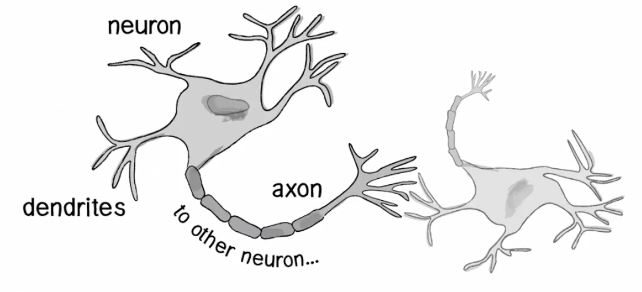Concept we are going to be trying to recreate through artificial neural networks (ANN).
- There are problems that are difficult by humans but easy for computers (calculating large arithmetic problems)
- Then there are problems easy for humans, but difficult for computer such as recognizing picture of a person and describing the images
- Neural networks attempt to solve problems that would normally be easy to humans but hard for computer.

Perceptron

A percepton consists of one or more inputs, a processor and single output.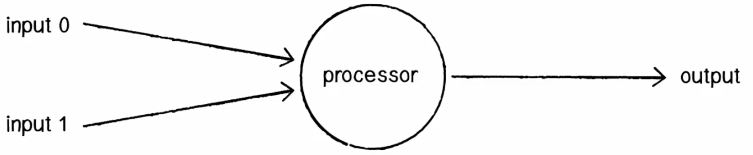Feed Forward

A perceptron follows the "feed-forward" model, meaning inputs are sent into the neuron, are processed and result in a output.
From this figure read from left to right, input 0 and input 1 going into the processor and then being output.

Weight

Each input that is sent into neuron must first be weighted it mean going to multiplied by some value (often a number between -1 and 1).
when creating a perceptron, we will typically begin by assigning random weights. We take the input and multiply it by its weight
Input 0 Weight 0 ==>12 * 0.5 = 6
Input 1 Weight 1 ==> 4 * -1 = -4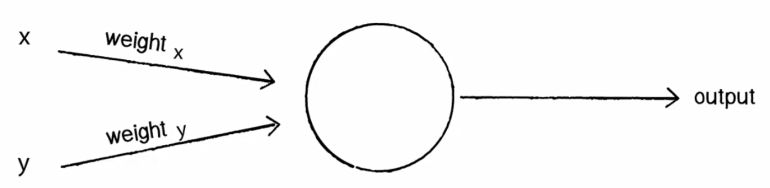Activation Function

The output of a perceptron is generated by passing that sum through an activation function. In the case of a simple binary output the activation function is what tells the perceptron whether to "fire" or not. There are many activation function like relu function, tanh function, step function.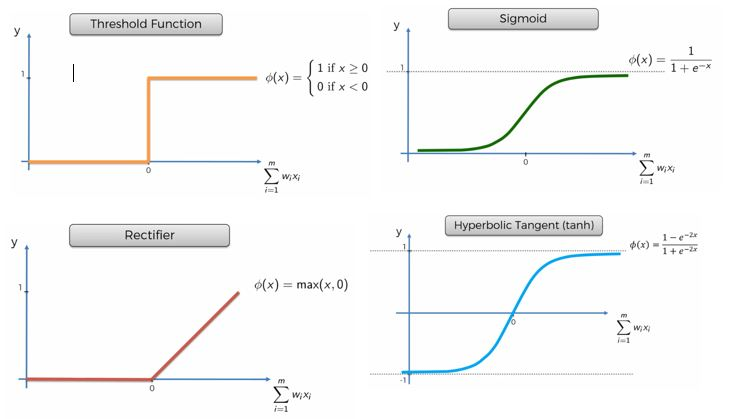Sigmoid function is very useful in the final layer output layer, especially when you are trying to predict probabilities
Rectifier function (RELu) is the most popular function for artificial neural network

Bias

Imagine that both inputs were equal to zero then any sum no matter what muliplicative weight would also be zero. It will be very common to have a point that may just happen on X is 0, Y is 0, in order to solve that problem we add bias.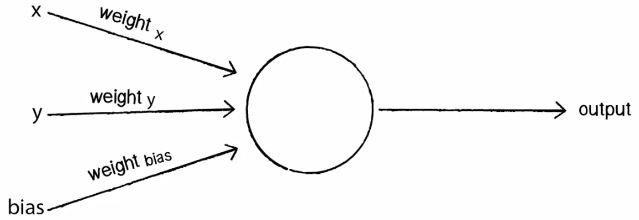To avoid this problem, we add a third input known as a bias input with a value of 1, this avoids the zero issues

Hidden Layer

You will have an input layer and an output layer. Any layers in between are known as hidden layers, because you don't directly "see" anything but the input or output.
You may heard of the term "Deep Learning", That is just a neural network with many hidden layers, causing it to be "Deep".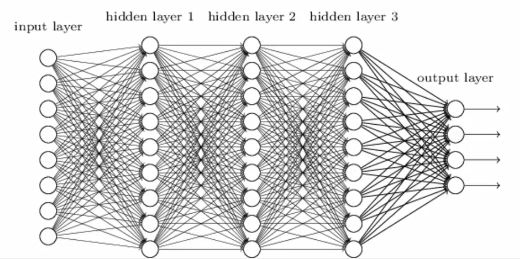Back Propagation

Best way to get fast understanding is with example, and there it is :
How do Neural Network learn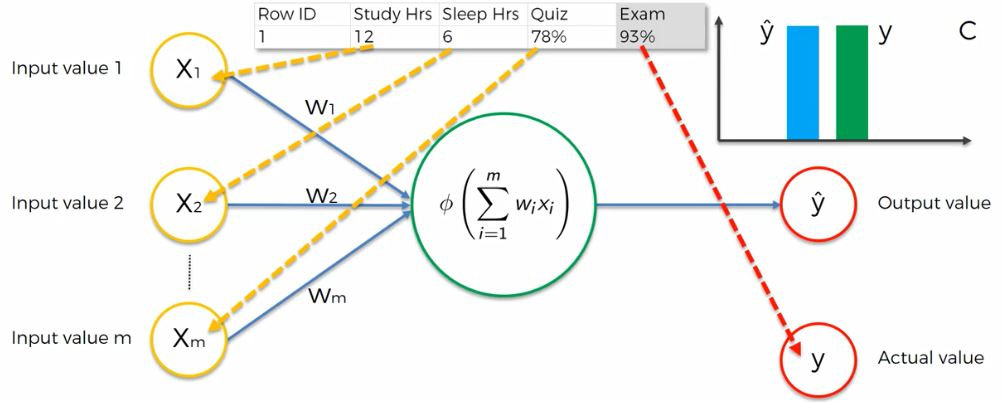We have 3 input layer :

X1 = Study_Hours
X2 = Sleep_Hours
x3 = Quiz

ŷ   = Output value (predicted value)
y = Actual value (real value)

C = Cost Function

So, we have some input values that have been supplied to the perceptron, then activation function is applied, then we have an output  ŷ.
Now we need to compare the output value  ŷ   to the actual value y.
After that we are going to calculated cost value, of course there is a bit of difference between actual value and output value.
Basically the cost function is telling us is what is the error that we have in our prediction.
Our Goal is to minimize the cost function because the lower cost function, the closer  ŷ   to y.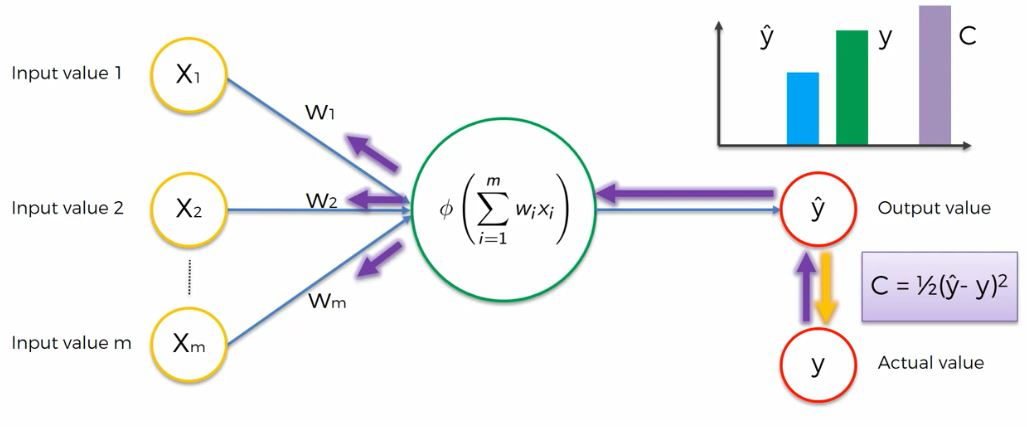Once we have compared, now we are going to feed this information back into the neural network and this called Back Propagation.
The weight get updated and this do repeatedly until minimize the cost function and to get as soon as found minimum cost function that is your final neural network that means your weights have been adjusted and you have found the optimal weights for this dataset.

Gradient Descent

https://iamtrask.github.io/2015/07/27/python-network-part2/

How can we minimized the cost function?
Well one approach to do it is a brute force approach, where we just take all lots of different possible weights and look at them and see which one looks best.
Let us say for example a thousand weights and we would try them out that would get something like this :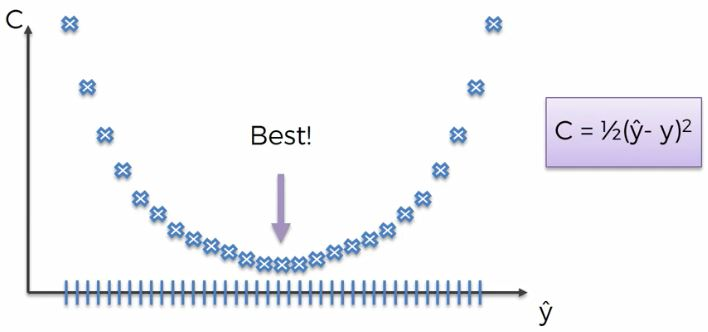So very simple, very intuitive approach.
Below is Gradient Descent algorithm visualization :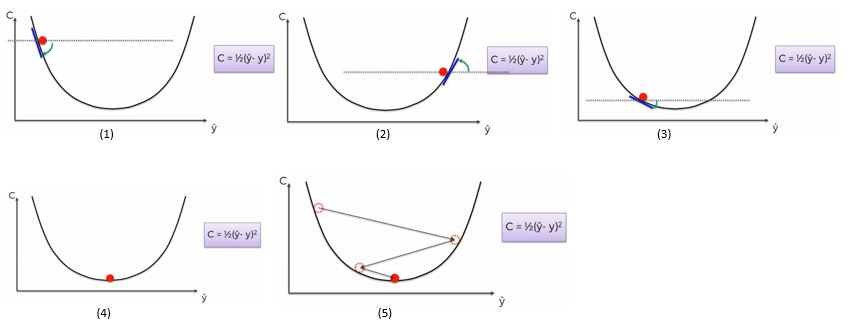Now we go into see how we can find a faster way to get the best option
start from point (1) we are going to look at the angle of our cost function at that poin so we are just going to basically what is called Gradient. If the slope is negative like in this case means you are going to the right to go downhill, the point (2) slope is positive so we go to left to go downhill and repeated point number (3) until found the best point (4).
Gradient descent is very efficient method to solve our optimization problem where we are trying to minimize the cost function. It basically takes us from years to solving a problem within minutes or hours and it really helps speed things up because we can see which way is downhill and we can just go in that direction and take steps and get to the minimum faster or until get slop = 0

Curse of Dimensionality

The best way to describe or explaining this is to just look at pratical example :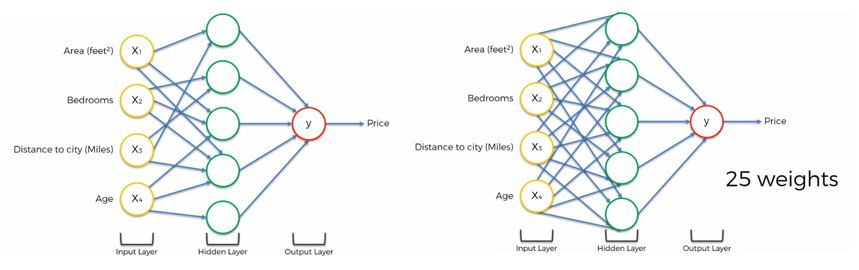Picture on the left is how neural networks actually work where we were building or running a neural network for a property valuation, so this is what looked like when it was trained up already.
Picture on the right is before we know which one what are the weights.
Let us see how we could possibly brute force 25 ways.
So, its give us very long long time if we use brute force approach.

Stochastic Gradient Descent

The weakness of gradient descent is that method requires for the cost function to be convex (curved or rounded outward like the exterior of a sphere or circle).
But what if cost function is not convex, have 2 or more global minimum? like picture bellow :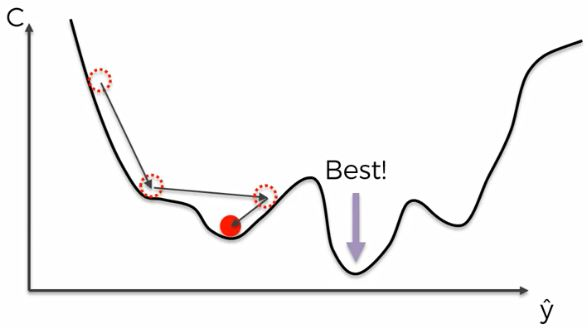In this case if we just tried to apply our normal gradient descent something that picture above could happen.
We could find a local minimum of the cost function rather than the global one. So this one was the best one and we found the wrong one and there we don't have correct weight. We don't have an optimized neural network.
So what we do in this case?
Well the answer is stochastic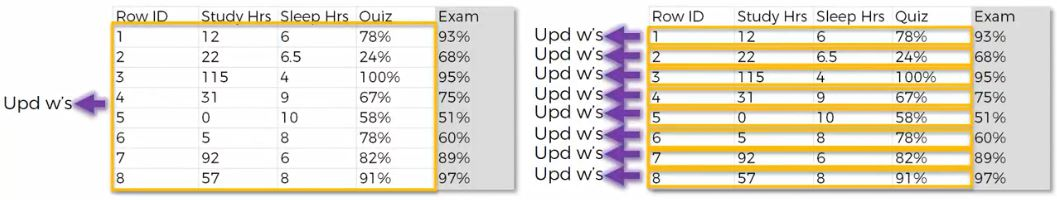Picture on right side is how SGD work, evaluate cost function for each rows and update weights
picture on left side is how DG work , evaluate cost function after all rows executes and update weights, and this method known as Batch Gradient Descent.